# ISEE Lower Level Quantitative : Geometry

## Example Questions

### Example Question #2 : Parallel Lines: Ccss.Math.Content.8.G.A.1c

Observe the location of the black and orange parallel lines on the provided coordinate plane and identify which of the following transformations—rotation, translation, or reflection—the black lines have undergone in order to reach the position of the orange lines. Select the answer that provides the correct transformation shown in the provided image.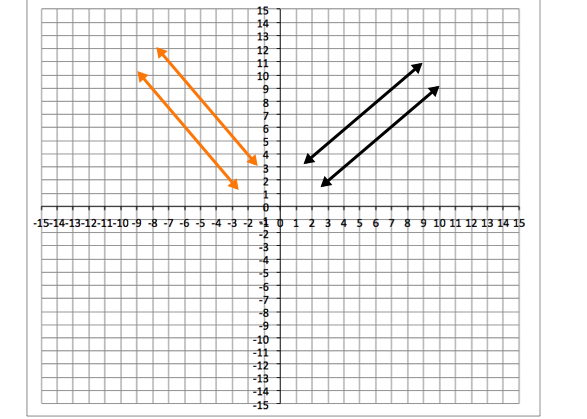Reflection over the y-axis

Translation to the left

A, clockwise rotation

Reflection over the y-axis

Explanation:

First, let's define the possible transformations.

Rotation: A rotation means turning an image, shape, line, etc. around a central point.

Translation: A translation means moving or sliding an image, shape, line, etc. over a plane.

Reflection: A reflection mean flipping an image, shape, line, etc. over a central line.

In the images from the question, the lines were not rotated, clockwise because that rotation would have caused the lines to move to the right, but the lines were moved to the right. The lines were not moved to the left, as the translation is described in the answer choice, because the lines changed direction; thus, the correct answer is a reflection over the y-axis.

### Example Question #1 : Parallel Lines: Ccss.Math.Content.8.G.A.1c

Observe the location of the black and orange parallel lines on the provided coordinate plane and identify which of the following transformations—rotation, translation, or reflection—the black lines have undergone in order to reach the position of the orange lines. Select the answer that provides the correct transformation shown in the provided image.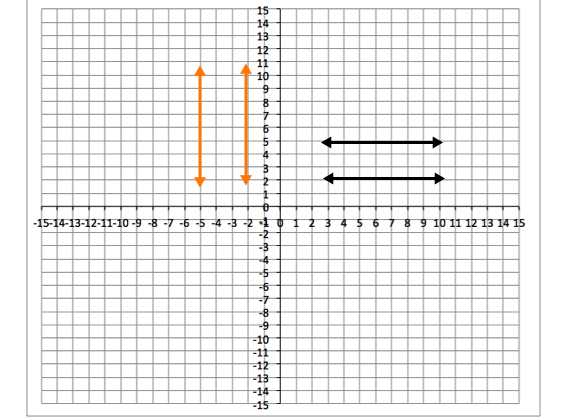Reflection over the x-axisrotation

Translation to the leftrotation

Explanation:

First, let's define the possible transformations.

Rotation: A rotation means turning an image, shape, line, etc. around a central point.

Translation: A translation means moving or sliding an image, shape, line, etc. over a plane.

Reflection: A reflection mean flipping an image, shape, line, etc. over a central line.

In the images from the question, notice that the lines made a rotation to the left around the y-axis, and the rotation was. Also, the lines changed from being horizontal to vertical, which is a sign that the lines were rotated; thus the transformation is a rotation.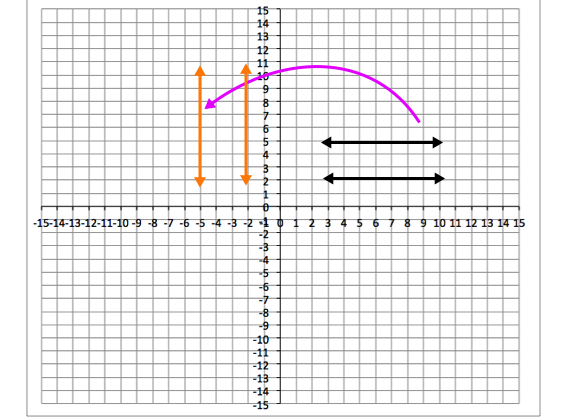The transformation can't be a reflection over the x-axis because the orange lines didn't flip over the x-axis.

The transformation can't be a translation because the line changes direction, which does not happened when you simply move or slide a line or image.

### Example Question #1 : Parallel Lines: Ccss.Math.Content.8.G.A.1c

Observe the location of the black and orange parallel lines on the provided coordinate plane and identify which of the following transformations—rotation, translation, or reflection—the black lines have undergone in order to reach the position of the orange lines. Select the answer that provides the correct transformation shown in the provided image.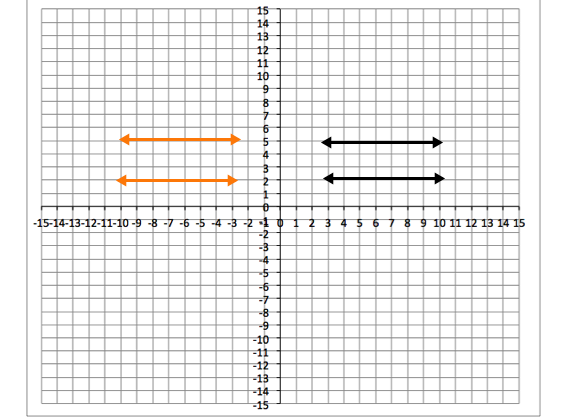A reflection over the x-axisrotation

A translation downrotation

Explanation:

First, let's define the possible transformations.

Rotation: A rotation means turning an image, shape, line, etc. around a central point.

Translation: A translation means moving or sliding an image, shape, line, etc. over a plane.

Reflection: A reflection mean flipping an image, shape, line, etc. over a central line.

In the images from the question, notice that the black lines rotates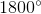counterclockwise, or left around the y-axis; thus the transformation is a rotation.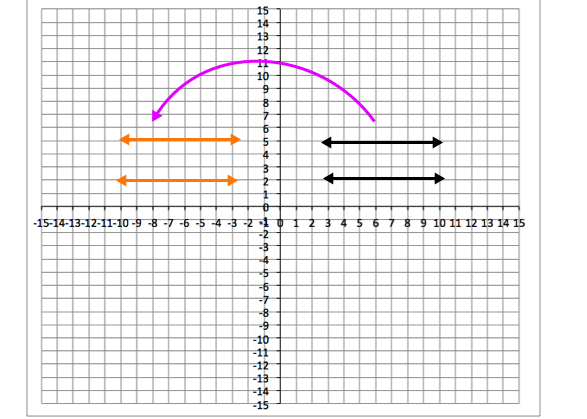The transformation can't be a reflection over the x-axis because the orange lines didn't flip over the x-axis.

The transformation can't be a translation down because the lines didn't not move down.

### Example Question #351 : Grade 8

Observe the location of the black and orange parallel lines on the provided coordinate plane and identify which of the following transformations—rotation, translation, or reflection—the black lines have undergone in order to reach the position of the orange lines. Select the answer that provides the correct transformation shown in the provided image.rotation

Translation to the left

A reflection over the x-axis

Translation to the left

Explanation:

First, let's define the possible transformations.

Rotation: A rotation means turning an image, shape, line, etc. around a central point.

Translation: A translation means moving or sliding an image, shape, line, etc. over a plane.

Reflection: A reflection mean flipping an image, shape, line, etc. over a central line.

In the images from the question, the lines were not rotatedbecause that rotation would have caused the lines to be vertical, but the lines are still horizontal. The lines were not reflected over the x-axis; thus the correct answer is a translation to the left.

### Example Question #1 : Parallel Lines: Ccss.Math.Content.8.G.A.1c

Observe the location of the black and orange parallel lines on the provided coordinate plane and identify which of the following transformations—rotation, translation, or reflection—the black lines have undergone in order to reach the position of the orange lines. Select the answer that provides the correct transformation shown in the provided image.rotation

A translation down and to the right

Reflection over the y-axis

Reflection over the y-axis

Explanation:

First, let's define the possible transformations.

Rotation: A rotation means turning an image, shape, line, etc. around a central point.

Translation: A translation means moving or sliding an image, shape, line, etc. over a plane.

Reflection: A reflection mean flipping an image, shape, line, etc. over a central line.

In the images from the question, the lines were not rotatedbecause that rotation would have caused the lines to be vertical, but the lines are still horizontal. The line was not moved down and to the right, as the translation is described in the answer choice; thus, the correct answer is a reflection over the y-axis.

### Example Question #31 : Geometry

Observe the location of the black and orange parallel lines on the provided coordinate plane and identify which of the following transformations—rotation, translation, or reflection—the black lines have undergone in order to reach the position of the orange lines. Select the answer that provides the correct transformation shown in the provided image.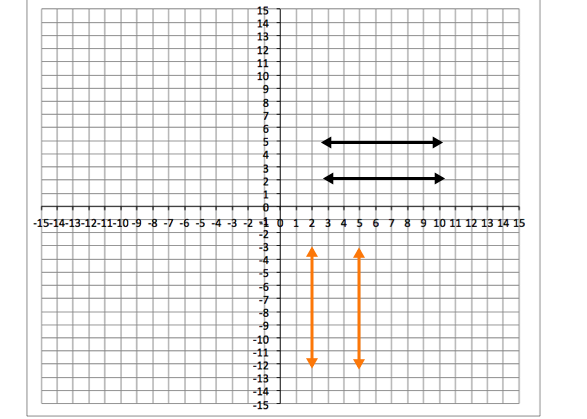A translation downrotation

A reflection over the y-axisrotation

Explanation:

First, let's define the possible transformations.

Rotation: A rotation means turning an image, shape, line, etc. around a central point.

Translation: A translation means moving or sliding an image, shape, line, etc. over a plane.

Reflection: A reflection mean flipping an image, shape, line, etc. over a central line.

In the images from the question, notice that the lines made a rotation to the right around the x-axis, and the rotation was; thus the transformation is a rotation.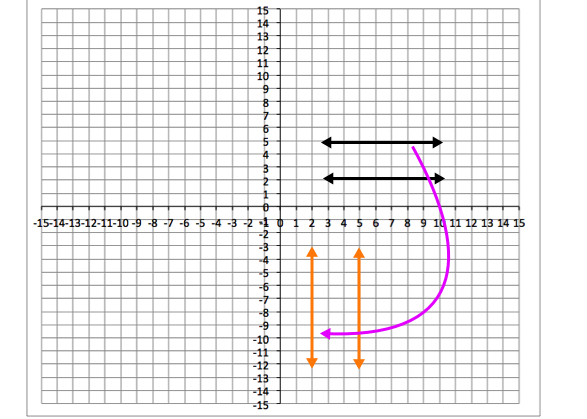The transformation can't be a reflection over the y-axis because the orange lines didn't flip over the y-axis.

The transformation can't be a translation because the lines changed direction, which does not happened when you simply move or slide a line or image.

### Example Question #861 : Geometry

Observe the location of the black and orange parallel lines on the provided coordinate plane and identify which of the following transformations—rotation, translation, or reflection—the black lines have undergone in order to reach the position of the orange lines. Select the answer that provides the correct transformation shown in the provided image.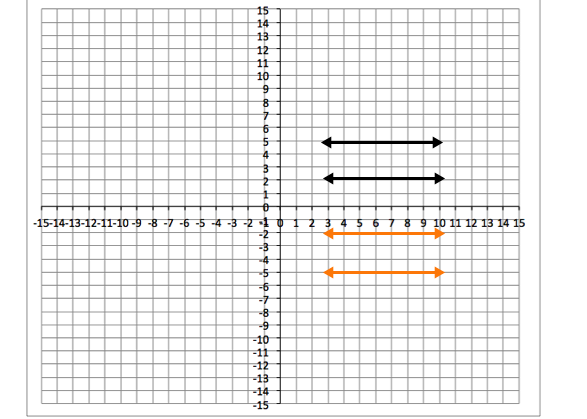A translation to the leftrotation

Reflection over the x-axis

Reflection over the x-axis

Explanation:

First, let's define the possible transformations.

Rotation: A rotation means turning an image, shape, line, etc. around a central point.

Translation: A translation means moving or sliding an image, shape, line, etc. over a plane.

Reflection: A reflection mean flipping an image, shape, line, etc. over a central line.

In the images from the question, the lines were not rotatedbecause that rotation would have caused the line to be vertical, but the line is still horizontal. The line was not moved to the left, as the translation is described in the answer choice; thus, the correct answer is a reflection over the x-axis.

### Example Question #861 : Geometry

Observe the location of the black and orange parallel lines on the provided coordinate plane and identify which of the following transformations—rotation, translation, or reflection—the black lines have undergone in order to reach the position of the orange lines. Select the answer that provides the correct transformation shown in the provided image.rotation

Translation down

Reflection over the y-axis

Translation down

Explanation:

First, let's define the possible transformations.

Rotation: A rotation means turning an image, shape, line, etc. around a central point.

Translation: A translation means moving or sliding an image, shape, line, etc. over a plane.

Reflection: A reflection mean flipping an image, shape, line, etc. over a central line.

In the images from the question, the lines were not rotatedbecause that rotation would have caused the lines to be vertical, but the lines are still horizontal. The lines were not reflected over the y-axis because that transformation would have caused the orange lines to be in the top left quadrant; thus, the correct answer is a translation down.

### Example Question #1 : Parallel Lines: Ccss.Math.Content.8.G.A.1c

Observe the location of the black and orange parallel lines on the provided coordinate plane and identify which of the following transformations—rotation, translation, or reflection—the black lines have undergone in order to reach the position of the orange lines. Select the answer that provides the correct transformation shown in the provided image.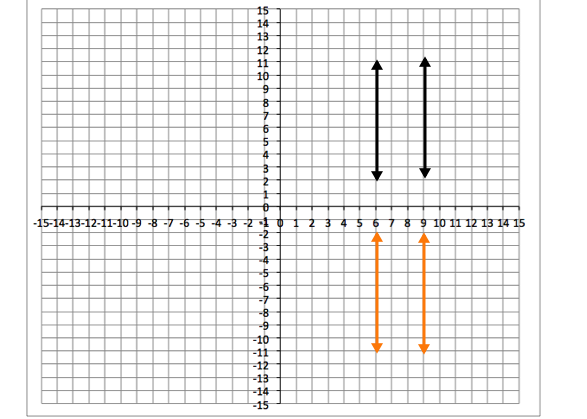Reflection over the x-axis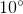rotation

A translation to the left

Reflection over the x-axis

Explanation:

First, let's define the possible transformations.

Rotation: A rotation means turning an image, shape, line, etc. around a central point.

Translation: A translation means moving or sliding an image, shape, line, etc. over a plane.

Reflection: A reflection mean flipping an image, shape, line, etc. over a central line.

In the images from the question, the lines were not rotatedbecause that rotation would have moved the lines to aslant, not straight. The line was not moved to the left, as the translation is described in the answer choice; thus, the correct answer is a reflection over the x-axis.

### Example Question #861 : Geometry

Observe the location of the black and orange parallel lines on the provided coordinate plane and identify which of the following transformations—rotation, translation, or reflection—the black lines have undergone in order to reach the position of the orange lines. Select the answer that provides the correct transformation shown in the provided image.rotation

Translation down

Reflection over the y-axis

Translation down

Explanation:

First, let's define the possible transformations.

Rotation: A rotation means turning an image, shape, line, etc. around a central point.

Translation: A translation means moving or sliding an image, shape, line, etc. over a plane.

Reflection: A reflection mean flipping an image, shape, line, etc. over a central line.

In the images from the question, the lines were not rotatedbecause that rotation would have caused the lines to be horizontal, but the lines are still vertical. The lines were not reflected over the y-axis because that transformation would have caused the orange lines to be in the top left quadrant; thus, the correct answer is a translation down.

### All ISEE Lower Level Quantitative Resources